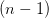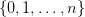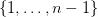# 2015-03-17 Nonlinear Seminar

Piotr Maćkowiak
A combinatorial lemma ant it's consequences

During this seminar we shall introduce a combinatorial lemma stating the existence for particular triangulations of the-dimensional simplex with a particular labeling of vertices of such triangulations with numbersof a chain of simplices connecting a "vartex" with a "base" such that for any simplex from that chain each element of the setis a label of some vertex of that simplex. We shall show, that (simple) consequences of this lemma are:

1. the existence of zeros of the multidimensional function of excess demand
2. the existence of continuum zeros of the parametrised multidimensional function of excess demand
3. the well-known Sperner lemma